# Inserting equations into Open edX

Mathematical equations are something that many specialist courses need, and thankfully it's not difficult to insert them into Open edX. In this article, we'll look at the basic steps to get your equation into your course content.

Please note: This article contains examples of the markup needed to use this feature. If you copy and paste examples from this article without pasting as plain text, you will likely paste extra formatting from our article along with the text and break this functionality. Please ensure you either paste as plain text or type it yourself, or it won't work!

First things first, you need to construct your equation. This is written in the common notation and document preparation language, LaTex, but if you're not familiar there are a variety of tools online you can use to construct your equation in a visual way. The one we've used most recently is this simple equation editor, but there are many others out there. We're just going to use it for this example, and the first thing we do once our equation is complete is export our equation out as LaTex: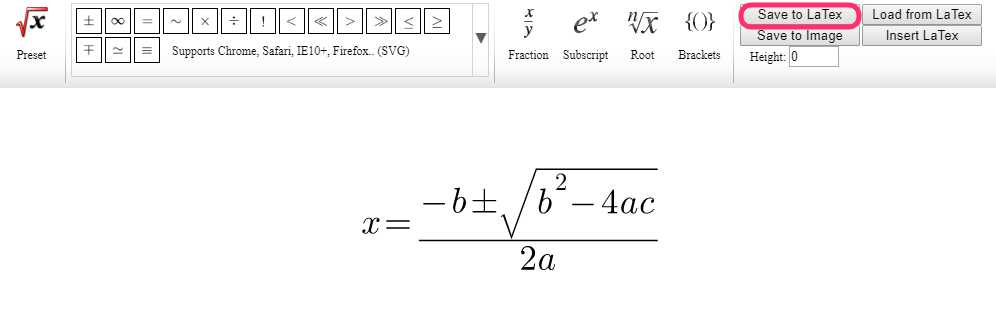When you have your LaTex, it'll end up looking something like this:

	{x=\frac {-b\pm \sqrt {{b}^{2}-4ac}} {2a}}


Now all we need to do is get it into the platform. First, find your HTML text component that you want to insert it into, or create a new one: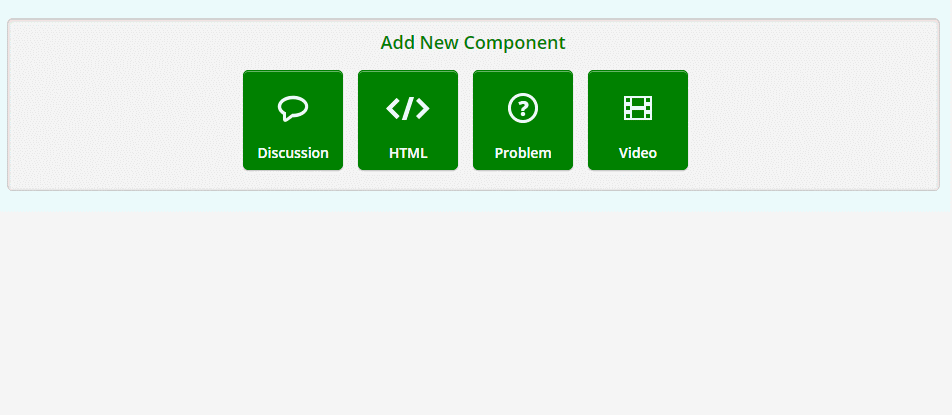Next, simply paste in your LaTex and surround it with the following:

	[mathjax][/mathjax]


So in this case:

	[mathjax]{x=\frac {-b\pm \sqrt {{b}^{2}-4ac}} {2a}}[/mathjax]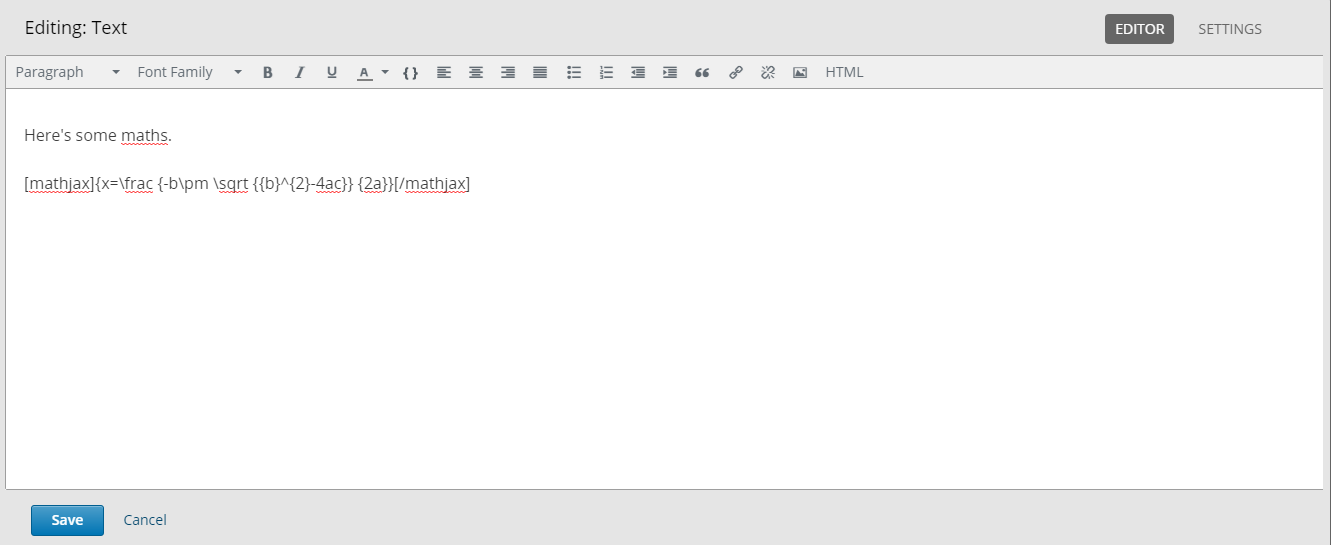Mathjax is what we use to turn that LaTex into proper mathematical notation. So with the above in place, you'll get something that looks like this once you hit Save: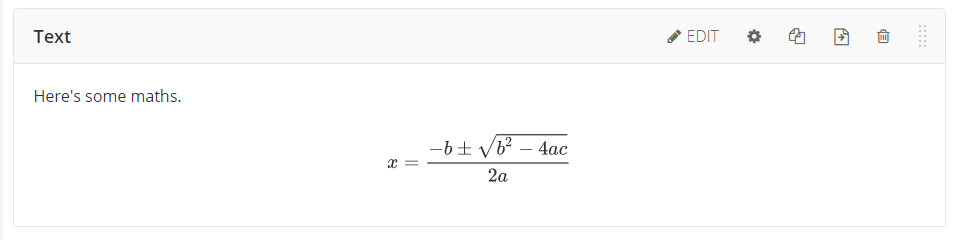If you want to put your equation in line with other text, you amend the code to [mathjaxinline] rather than [mathjax], for example:

	This fraction is inline with my text [mathjaxinline]\frac {1}{4}[/mathjaxinline] so that text goes on either side of it.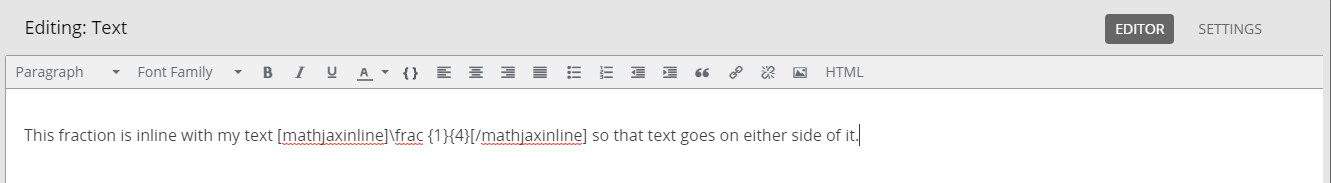This displays the equation or figure inline with the rest of your text.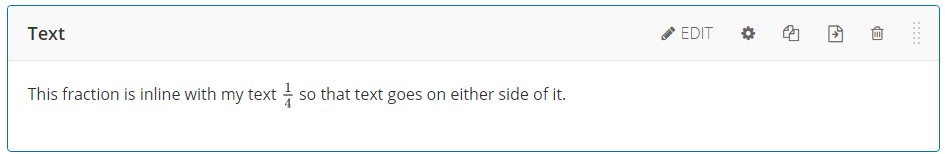Armed with this knowledge, you should now be able to go forth and speak the universal language of mathematics. As always, remember to Save and Publish your work!